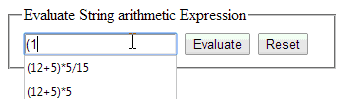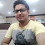### How to evaluate mathematical/arithmetic string expression in asp.net C# and VBIntroduction: In this article i am going to explain how to evaluate/solve the arithmetic/mathematical expression/formula which is in string form in asp.net using both C# and VB language. In previous articles i explained How to get age in years,months,days,hours and seconds from DOB and Convert Rupees,Currency or Numbers to Words and Get Title,Description and Keywords Meta tags from URL and Show tool tip message using CSS and HTML and Prevent duplicate record entry on page refresh and Turn off browser autocomplete feature in TextBox

Description: While working on asp.net project it was required to evaluate the arithmetic expression that was contained in string form.  As the formula was in string form, so direct evaluation was not possible.  After searching the internet for better solution i got a trick to use datatable's compute method to evaluate the string equations and my problem was solved. So i decided to create an article to share that trick with other developers so that it can help them also. I explored the compute method of data table thoroughly and created a function that can evaluate string arithmetic expressions.

Implementation: Let's create a demo website page to solve the arithmetic formula contained in string format.

ASP.NET C# Section:
• In asp.net design page (.aspx) place a textbox, label and a button controls as:
<fieldset style=" width:300px">
<legend>Evaluate String arithmetic Expression</legend>
<asp:TextBox ID="txtEquation" runat="server"></asp:TextBox>
<asp:Button ID="btnEvaluate" runat="server" Text="Evaluate"
onclick="btnEvaluate_Click" />

<asp:Button ID="btnReset" runat="server" Text="Reset"
onclick="btnReset_Click" />
<br />
<asp:Label ID="lblResult" runat="server" Text=""></asp:Label>
</fieldset>

ASP.NET C# Code to evaluate string form arithmetic expression
• In the code behind file (.aspx.cs) write the code as:
First include following required namespaces:
using System.Data;
using System.Drawing;

Now the most important is to create a function that can evaluate arithmetic expression which is in string form as.

private object EvaluateExpression(string eqn)
{
DataTable dt = new DataTable();
var result = dt.Compute(eqn, string.Empty);
return result;
}

Now call the above created function and pass the string equation that you want to evaluate to that function on Evaluate button click event as:

protected void btnEvaluate_Click(object sender, EventArgs e)
{
try
{
string result = Convert.ToString(EvaluateExpression(txtEquation.Text.Trim()));
lblResult.Text = "Result: " + result;
lblResult.ForeColor = Color.Green;
}
catch (Exception ex)
{
lblResult.Text = "Oops!! error occured: " + ex.Message.ToString();
lblResult.ForeColor = Color.Red;
}
}

protected void btnReset_Click(object sender, EventArgs e)
{
txtEquation.Text = string.Empty;
txtEquation.Focus();
lblResult.Text = string.Empty;
}

ASP.NET VB Section:
• In asp.net design page (.aspx) place a textbox, label and a button controls as:
<fieldset style=" width:300px">
<legend>Evaluate String arithmetic Expression</legend>
<asp:TextBox ID="txtEquation" runat="server"></asp:TextBox>
<asp:Button ID="btnEvaluate" runat="server" Text="Evaluate"/>

<asp:Button ID="btnReset" runat="server" Text="Reset"/>
<br />
<asp:Label ID="lblResult" runat="server" Text=""></asp:Label>
</fieldset>

ASP.NET VB Code to evaluate string form arithmetic expression
• In the code behind file (.aspx.vb) write the code as:
First import the following required namespaces:
Imports System.Data
Imports System.Drawing

Now the most important is to create a function that can evaluate arithmetic expression which is in string form as.

Private Function EvaluateExpression(eqn As String) As Object
Dim dt As New DataTable()
Dim result = dt.Compute(eqn, String.Empty)
Return result
End Function

Now call the above created function and pass the string equation that you want to evaluate to that function on Evaluate button click event as:

Protected Sub btnEvaluate_Click(sender As Object, e As System.EventArgs) Handles btnEvaluate.Click
Try
Dim result As String = Convert.ToString(EvaluateExpression(txtEquation.Text.Trim()))
lblResult.Text = "Result: " & result
lblResult.ForeColor = Color.Green
Catch ex As Exception
lblResult.Text = "Oops!! error occured: " & ex.Message.ToString()
lblResult.ForeColor = Color.Red
End Try
End Sub

Protected Sub btnReset_Click(sender As Object, e As System.EventArgs) Handles btnReset.Click
txtEquation.Text = String.Empty
txtEquation.Focus()
lblResult.Text = String.Empty
End Sub

Now over to you:
" I hope you have got what the trick to solve the string form arithmetic formulas in Asp.Net MVC and If you like my work; you can appreciate by leaving your comments, hitting Facebook like button, following on Google+, Twitter, Linked in and Pinterest, stumbling my posts on stumble upon and subscribing for receiving free updates directly to your inbox . Stay tuned and stay connected for more technical updates."
Previous
Next Post »

Intel

Good one sir ji ...:-PMike

I get my problem solved as i believe to hire asp.net professionals to get rid from such problems, but what is all about the experience.Anonymous